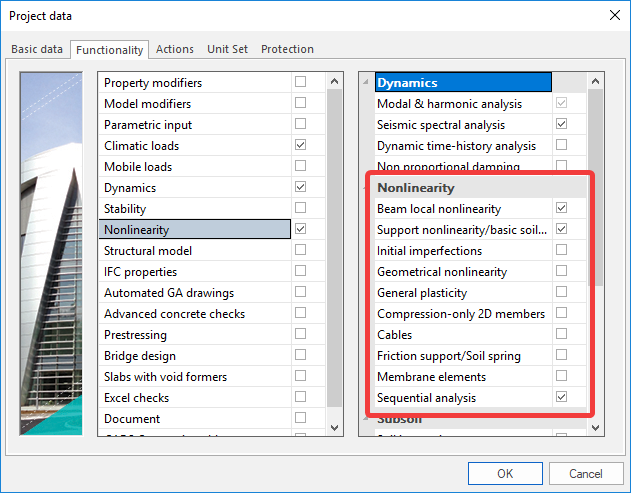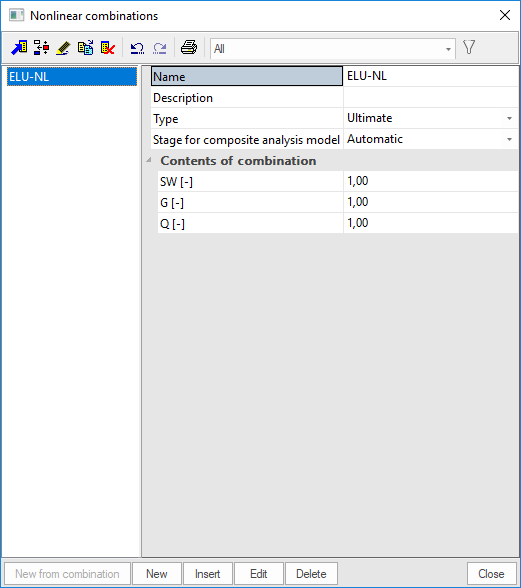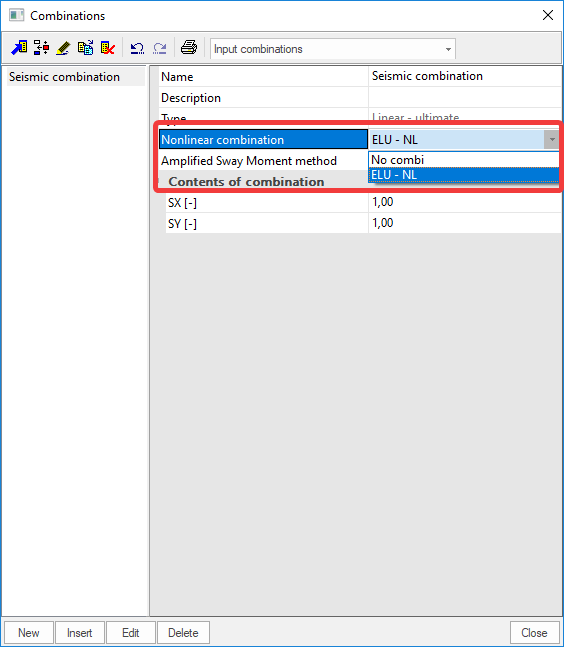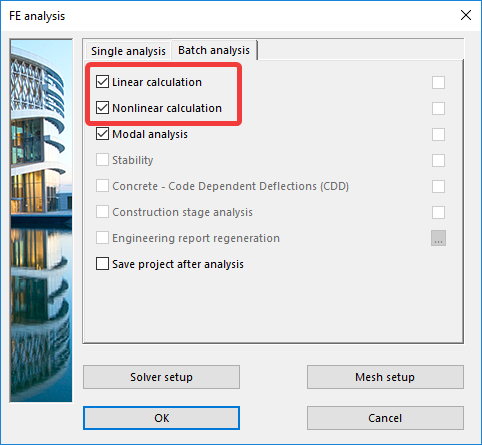# How to combine nonlinear combinations with seismicity

Seismic calculation is always a linear calculation. However, you can combine the seismic load cases with a nonlinear combination.

To do this, you will need to make a nonlinear combination (excluding the seismic load cases) and use these results in your linear seismic combination. In other words, the nonlinear behavior of the structure for the static forces will be combined with the linear seismic calculation.

Step 1: Create all needed load cases including the seismic load cases.

Step 2: Add all needed nonlinearities to the structure. Make sure the necessary nonlinear functionalities are activated.Step 3: Create a nonlinear combination containing all required load cases except the seismic load cases. As mentioned above, the seismic calculation is only linear.Step 4: Create a linear combination containing only the seismic load cases. In your linear combination, choose the nonlinear combination that was created in step 3.Step 5: Run the batch analysis.Remark: Using this procedure the combination should be defined manually meaning SCIA will not calculate the coefficient automatically for each load case.